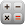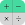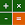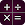# Calculator PRO - Free Scientific Equation Solver

by Express Dev Inc
500,000+ installs

Android application Calculator PRO - Free Scientific Equation Solver developed by is listed under category Productivity. According to Google Play Calculator PRO - Free Scientific Equation Solver achieved more than 500,000 installs. Calculator PRO - Free Scientific Equation Solver currently has 9,775 ratings with average rating value of 4.699. The current percentage of ratings achieved in last 30 days is 3.18%, percentage of ratings achieved in last 60 days is 4.78%. Calculator PRO - Free Scientific Equation Solver has the current market position #31643 by number of ratings. A sample of the market history data for Calculator PRO - Free Scientific Equation Solver can be found below. Last update on 2019-09-01.

Title: Calculator PRO - Free Scientific Equation Solver Express Dev Inc Productivity Free Android
Total ratings: 9775 3.18% 4.78% 4.699
Installs (achieved): 500,000+ 797,959
5 star ratings: 8,364 701 250 100 360

### Screenshots

2019-05-02: Android application Calculator PRO - Free Scientific Equation Solver achieved 500,000 installs. Android application Calculator PRO - Free Scientific Equation Solver achieved 100,000 installs. Android application Calculator PRO - Free Scientific Equation Solver achieved 10,000 installs.

Total number of ratings
Total number of active users rated for Calculator PRO - Free Scientific Equation Solver.

Total number of installs (*estimated)
Estimation of total number of installs on Google Play. Approximated from number of ratings and install bounds achieved on Google Play.

Average rating
Average rating value on Google Play. Given by active users of the application.